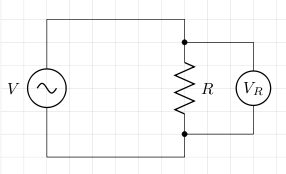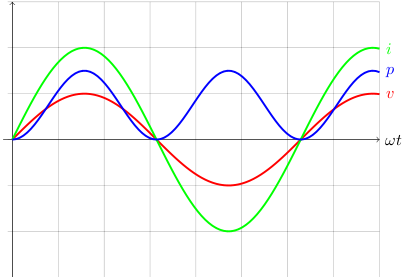# Resistance (AC)

Consider the following circuit:From what we know about AC voltage, the instantaneous voltage across the resistor is

```VR = V0sin(ωt)
```

where ω is the angular frequency. If the instantaneous voltage is v, then the instantaneous current, i, is

```i = v/R
= V0sin(ωt)/R
```

The maximum current, I0, is when sin(ωt)=1, so

```I0 = V0/R
```

So,

```i = I0sin(ωt)
```

If p is instantaneous power, and P0 is maximum power, then

```p = iv
= i2R
= (I0sin(ωt))2R
= I02R sin2(ωt)
P0 = I02R
p = P0sin2(ωt)
```

The following graph shows the relationship between the instantaneous values of voltage (v), current (i), and power (p):## References

Fischer-Cripps. A.C., The Electronics Companion. Institute of Physics, 2005.